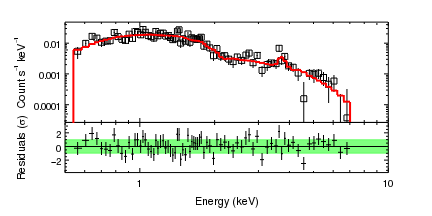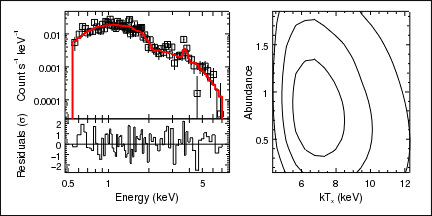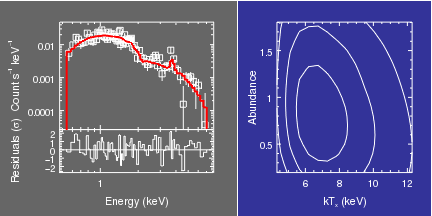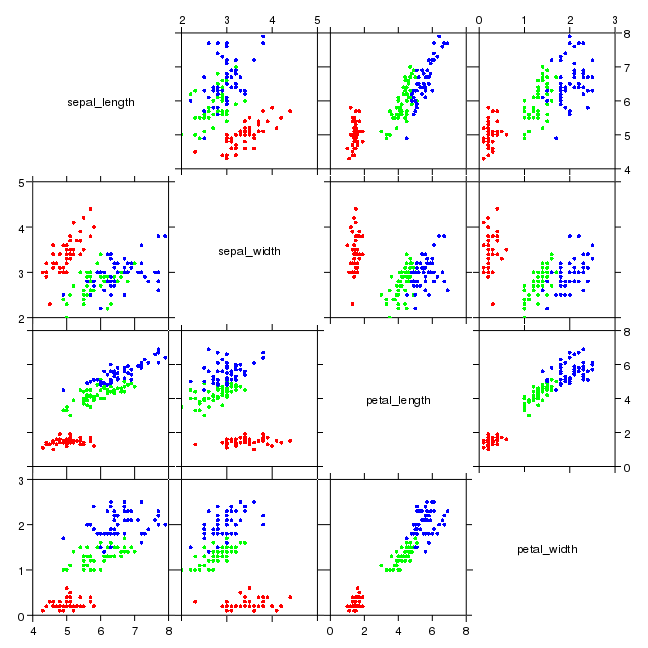# Gallery: Multiple plots

## 1) Data, fit, and residuals

This visualization contains two plots arranged vertically, with multiple curves in the first plot. It makes use of the strip_chart command to automatically create multiple plots with "shared" axes (so that a change to the limits of the X axis of one plot is automatically reflected in the other plot).Version: Postscript; PDF
set_preferences(["axis.offset.perpendicular",50,"plot.bottommargin",0.2])

strip_chart(2)

crv = ChipsCurve()
crv.line.style = "noline"
crv.symbol.style = "square"
crv.symbol.fill = False
crv.symbol.size = 3

hist = ChipsHistogram()
hist.line.color = "red"
hist.line.thickness = 2

log_scale(Y_AXIS)
set_plot_ylabel("Count s^{-1} keV^{-1}")

current_plot("plot2")

x = copy_colvals(resid,"x")
y = copy_colvals(resid,"y")
dylo = copy_colvals(resid,"dylo")
dyhi = copy_colvals(resid,"dyhi")
dxlo = copy_colvals(resid,"dxlo")
dxhi = copy_colvals(resid,"dxhi")
set_curve(["line.style","noline","symbol.style","none"])

log_scale(X_AXIS)
limits(X_AXIS,0.5,10)

set_plot_xlabel("Energy (keV)")
set_plot_ylabel(r"Residuals (\sigma)")

set_xaxis(["offset.perpendicular",40])

# Add a region highlighting the +/- 1 sigma region
xr = [0.5, 10, 10, 0.5]
yr = [-1, -1, 1, 1]
shuffle_region(chips_back)


The set_preference call is used since we know that we need to increase the spacing of the labels for the Y axes of these plots. The offset.perpendicular setting could instead have been changed after the plots were created. A window is explicitly created using add_window so that the dimensions can be changed from the default values; in this case we want a rectangular area that is wider than it is tall (the default window size is square).

Since we want two plots arranged vertically, we can just call strip_chart with a single argument of 2. This creates two equally-sized plots and makes the top plot the current one (i.e. any data we plot such as a curve or histogram will appear in the top plot).

We add a curve and then a histogram to this top plot, using add_curve and add_histogram, and change the attributes of these objects. Before moving to the second plot, the Y axis is changed to a logarithmic scale and a label added to it.

The current_plot call changes to the second plot (the info and info_current commands are useful for finding out what ChIPS objects have been created and what there names are). In order to plot the data - which consists of (x,y) points with errors on both axes - we need to use arrays rather than a filename in the add_curve call. The column values are read in using crates routines; read_file to read in the file and copy_colvals to get the column values. As we only want the errors to be displayed we set the line style of the curve to "noline" and the symbol style to "none".

add_axis(X_AXIS, 0, 0, 1)
bind_axes("ax1", "ax2")


but the extra information of axes, such as the tick marks and labels, obscure some of the information in this case.

The log_scale and limits calls change the X axes of both plots, since they have been bound together by the strip_chart call.

The relative sizes of the two plots are changed by the adjust_grid_yrelsize call, which makes the first row twice as high as any other row (in this case there is only a single column and two rows).

A region is used to highlight the 1-sigma range for the residuals; it is moved behind the points using the shuffle_region command so that it does not obscure the points in the PS output.

The output of the info and info_current commands is shown below:

chips> info()

Window [win1]
Frame [frm1]
Plot [plot1]   (0.15,0.43)  .. (0.90,0.90)
Border bottom [bx1]  top [bx2]  left [by1]  right [by2]
X Axis [ax1]
Y Axis [ay1]
Curve [crv1]
Histogram [hist1]
Plot [plot2]   (0.15,0.20)  .. (0.90,0.43)
Border bottom [bx1]  top [bx2]  left [by1]  right [by2]
X Axis [ax1]
Y Axis [ay1]
Curve [crv1]
Line [line1]
Region [reg1]

chips> info_current()

Window [win1]
Frame [frm1]
Plot [plot2]
X Axis [ax1]
Y Axis [ay1]
Curve [crv1]
Line [line1]
Region [reg1]
Coord Sys [Data]
Coord Sys ID [ds0.0.1.5]



## 2) Data, residuals, and contours in one frame

In this example we take the data plotted in the first example and add an extra plot, showing the confidence contours of the fit for two of the parameters. All the plots are placed in the same frame; the layout is created by the low-level col_grid_objects routine which allows you to create a different number of plots in each column. See the following example for a different way of plotting the same data.Version: Postscript; PDF
pref = ChipsPreferences()
pref.frame.border.visible = True
pref.axis.offset.perpendicular = 50
pref.plot.bottommargin = 0.2
pref.plot.rightmargin = 0.05
pref.plot.topmargin = 0.05
set_preferences(pref)

col_grid_objects([2,1],0.1,0,1)

crv = ChipsCurve()
crv.line.style = "noline"
crv.symbol.style = "square"
crv.symbol.fill = False
crv.symbol.size = 3
set_curve(crv)

hist = ChipsHistogram()
hist.line.color = "red"
hist.line.thickness = 2

log_scale(Y_AXIS)
set_plot_ylabel("Count s^{-1} keV^{-1}")
set_xaxis("all",["ticklabel.visible",False,"minortick.visible",False])
set_plot(["bottommargin",0.45])

current_plot("plot2")

set_plot(["topmargin",0.55])

set_plot_xlabel("Energy (keV)")
set_plot_ylabel(r"Residuals (\sigma)")

set_xaxis(["offset.perpendicular",35])

bind_axes("plot1","ax1","plot2","ax1")
log_scale(X_AXIS)

# Set labels along the X axis
set_xaxis("all",["minortick.visible",False])
x = [0.5, 0.6, 0.7, 0.8, 0.9, 1, 2, 3, 4, 5, 6, 7]
xl = [0.5, "", "", "", "", 1, "", "", "", 5, "", ""]
set_arbitrary_tick_positions("ax1",x,xl)

current_plot("plot3")

set_plot_xlabel("kT_x (keV)")
set_plot_ylabel("Abundance")

set_xaxis(["offset.perpendicular",35])
set_yaxis(["offset.perpendicular",25])


In general the commands used are similar to the first example, although here we have to manually create a frame using add_frame; col_grid_objects is unlike most ChIPS commands in that it will not automatically create the parent objects for you. The frame border has been turned on for comparison with the multi-frame example below.

Once the plots have been created the data can be plotted as before; although note that the residuals are this time plotted as a histogram (without error bars) rather than a curve; the X axis labels of the first plot have been hidden using the ticklabel.visible attribute of the axis; and that the X axes of the data and residual plots have to be manually linked together using the bind_axes call.

The log-axis display in ChIPS only labels the major tick marks, which can make the axis hard to read when the range is only a factor of 10. We therefore use the set_arbitrary_tick_positions command to add tick marks at x=0.5, 0.6, 0.7, 0.8, 0.9, 1, 2, 3, 4, 5, 6, 7 and labels at x=0.5, 1, 5. The minor tick marks are turned off in this plot and in "plot1" as they are not useful in this case.

The output of the info and info_current commands is shown below:

chips> info()

Window [win1]
Frame [frm1]
Plot [plot1]   (0.15,0.45)  .. (0.53,0.95)
Border bottom [bx1]  top [bx2]  left [by1]  right [by2]
Curve [crv1]
X Axis [ax1]
Y Axis [ay1]
Histogram [hist1]
Plot [plot2]   (0.15,0.20)  .. (0.53,0.45)
Border bottom [bx1]  top [bx2]  left [by1]  right [by2]
X Axis [ax1]
Y Axis [ay1]
Histogram [hist1]
Line [line1]
Plot [plot3]   (0.63,0.20)  .. (0.95,0.95)
Border bottom [bx1]  top [bx2]  left [by1]  right [by2]
Contour [ctr1]
X Axis [ax1]
Y Axis [ay1]

chips> info_current()

Window [win1]
Frame [frm1]
Plot [plot3]
Contour [ctr1]
X Axis [ax1]
Y Axis [ay1]
Coord Sys [Data]
Coord Sys ID [ds0.0.2.10]



## 3) Data and residuals in one frame, contours in another

This example shows how the data used in the previous example can be plotted using multiple frames: the fit and residuals plots are drawn in one frame and the contour plot added to a second frame.Version: Postscript; PDF
add_window(6,3,"inches")
strip_chart(2)
reposition_frame(0,0,0.55,1)
set_frame(["bgcolor","666666"])

crv = ChipsCurve()
crv.line.style = "noline"
crv.symbol.style = "square"
crv.symbol.fill = False
crv.symbol.size = 3
set_curve(crv)

hist = ChipsHistogram()
hist.line.color = "red"
hist.line.thickness = 2

log_scale(Y_AXIS)
set_plot_ylabel("Count s^{-1} keV^{-1}")
set_xaxis(["ticklabel.visible",False])
set_plot(["bottommargin",0.45])

current_plot("plot2")

log_scale(X_AXIS)

set_plot_xlabel("Energy (keV)")
set_plot_ylabel(r"Residuals (\sigma)")

set_plot(["bottommargin",0.2])
set_plot("all",["leftmargin",0.25])
set_yaxis(["offset.perpendicular",50])

set_frame(["bgcolor","333399"])

set_plot_xlabel("kT_x (keV)")
set_plot_ylabel("Abundance")

set_plot(["leftmargin",0.2,"bottommargin",0.2])
set_yaxis(["offset.perpendicular",25])

set_preference("foreground.file","white")


By using two frames, some operations are simpler in this version, since we can now use strip_chart to plot the data from the file "spectrum.fits"; however we now have to manually position and re-size the frames within the window (using commands like reposition_frame and add_frame).

The output of the info and info_current commands is shown below:

chips> info()

Window [win1]
Frame [frm1]
Plot [plot1]   (0.25,0.40)  .. (0.90,0.90)
Border bottom [bx1]  top [bx2]  left [by1]  right [by2]
X Axis [ax1]
Y Axis [ay1]
Curve [crv1]
Histogram [hist1]
Plot [plot2]   (0.25,0.20)  .. (0.90,0.40)
Border bottom [bx1]  top [bx2]  left [by1]  right [by2]
X Axis [ax1]
Y Axis [ay1]
Histogram [hist1]
Line [line1]
Frame [frm2]
Plot [plot1]   (0.20,0.20)  .. (0.90,0.90)
Border bottom [bx1]  top [bx2]  left [by1]  right [by2]
Contour [ctr1]
X Axis [ax1]
Y Axis [ay1]

chips> info_current()

Window [win1]
Frame [frm2]
Plot [plot1]
Contour [ctr1]
X Axis [ax1]
Y Axis [ay1]
Coord Sys [Data]
Coord Sys ID [ds0.1.2.9]


## 4) A scatterplot of the Fisher Iris data set

The ChIPS split command can be used to make a grid of scatter plots, showing how a set of variables are related; that is, to create a scatterplot grid, or matrix, but it can be quite tiresome to plot all the data and set up the axes so that they are bound together. To simplify this, the scatterplots command, from the chips_contrib.scatter module has been added.

In this example the scatterplots routine is used to plot up the data from Fisher's Iris flower data set, showing possible correlations between the different species.Version: Postscript; PDF
# Load in scatterplot-related commands
from chips_contrib.scatter import *

# Plot the three species with different colors. The margins
# are reduced to make the grid fill the frame.
scatterplots('iris.fits[species=setosa]', color='red', margin=0.05)
scatterplots('iris.fits[species=versicolor]', color='green', overplot=True)
scatterplots('iris.fits[species=virginica]', color='blue', overplot=True)

# Adjust the gap between the plots; the gap argument to scatterplots
# could also have been used to set this value

# The default axis limits are set to try to minimize overlap,
# but they do not always make best use of the available space,
# so we change the limits used for the sepal_length and petal_length
# variables.
scatterlimits('petal_length', 0, 8)
scatterlimits('sepal_length', 4, 8)


For this example the data was download as a CSV file, iris.csv, and converted to FITS form using the ASCII support in the CIAO data model:

unix% head -3 iris.csv
species,sepal length,sepal width,petal length,petal width
setosa,5.1,3.5,1.4,0.2
setosa,4.9,3,1.4,0.2
unix% dmcopy "iris.csv[opt skip=1,sep=','][cols species=col1,sepal_length=col2, sepal_width=col3, petal_length=col4,petal_width=col5]" iris.fits
unix% dmlist iris.fits cols

--------------------------------------------------------------------------------
Columns for Table Block iris.csv
--------------------------------------------------------------------------------

ColNo  Name                 Unit        Type             Range
1   species                           String
2   sepal_length                      Real8          -Inf:+Inf
3   sepal_width                       Real8          -Inf:+Inf
4   petal_length                      Real8          -Inf:+Inf
5   petal_width                       Real8          -Inf:+Inf


The scatterplots command is used to plot up the three datasets - using a Data Model filter to select the three species - as the red, green, and blue circles. The adjust_grid_gaps call changes the spacing between the plots; the gap argument of scatterplots could also have been used, but that requires knowing the spacing before the visualization has been created.

To finish, the limits used to display the petal_length and sepal_length variables are changed with the scatterlimits command, since the default limits were too large.

For reference, the numeric values in the columns have the following ranges:

unix% dmstat iris.fits sigma- | egrep '_|min|max'
sepal_length
min:	4.3 	      @:	14
max:	7.9 	      @:	132
sepal_width
min:	2 	      @:	61
max:	4.4 	      @:	16
petal_length
min:	1 	      @:	23
max:	6.9 	      @:	119
petal_width
min:	0.1 	      @:	10
max:	2.5 	      @:	101